# Standard Enthalpy and Gibbs Free Energy of Reaction Notes | Study Additional Documents & Tests for Civil Engineering (CE) - Civil Engineering (CE)

## Civil Engineering (CE): Standard Enthalpy and Gibbs Free Energy of Reaction Notes | Study Additional Documents & Tests for Civil Engineering (CE) - Civil Engineering (CE)

The document Standard Enthalpy and Gibbs Free Energy of Reaction Notes | Study Additional Documents & Tests for Civil Engineering (CE) - Civil Engineering (CE) is a part of the Civil Engineering (CE) Course Additional Documents & Tests for Civil Engineering (CE).
All you need of Civil Engineering (CE) at this link: Civil Engineering (CE)

Standard Enthalpy and Gibbs free energy of reaction

From the foregoing discussion it may be apparent that a chemical reaction may be carried out in diverse ways by changing temperature, pressure, and feed composition. Each of the different conditions would involve different conversions and heat effects. Thus there is need to define a “standard” way of carrying out a reaction. If all reactions were carried out in the same standard manner, it becomes possible to compare them with respect to heat effects, and equilibrium conversion under the same conditions. In general all reactions are subject to heat effects, whether small or large. A reaction may either release heat (exothermic) or absorb heat (endothermic). However, it is expected that the heat effect will vary with temperature. Thus, there is a need to develop general relations that allow computation of the heat effect associated with a reaction at any temperature.

Consider a reaction of the following form: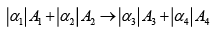.....(8.1)

The reactants (Aand A2 ) and products (A 3 and A4 ) may be gaseous, liquid or solid. The term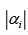is the stoichiometric coefficient corresponding to the chemical species Ai
For the purpose of development of the reaction equilibria relations it is convenient to designate the stoichiometric numbers of the reactants as negative, while those of the products as positive. This is to signify that reactants are depleted in proportion to their stoichiometric numbers, while the products are formed in proportion to their stoichiometric numbers. Consider, for example, the following gas-phase reaction: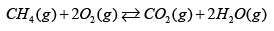The stoichiometric numbers are written as follows: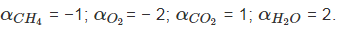The standard enthalpy of reaction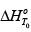at say at any temperature T is defined in the following manner: it is the change in enthalpy that occurs whenmoles of Aand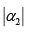moles of B2 in their standard states at temperature T convert fully to form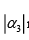moles of A 3 and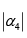moles of A4 in their respective standard states at the same temperature T. The standard states commonly employed are as

— Gases: the pure substance in the ideal gas state at 1 bar

— Liquids and Solids: the pure liquid or solid at 1 bar

The conceptual schema of a standard reaction is depicted in fig. 8.1. All reactants enter and products leave the reactor in pure component form at the same temperature T, and at their respective standard states. In the literature, data on the standard enthalpy of reaction is typically reported at a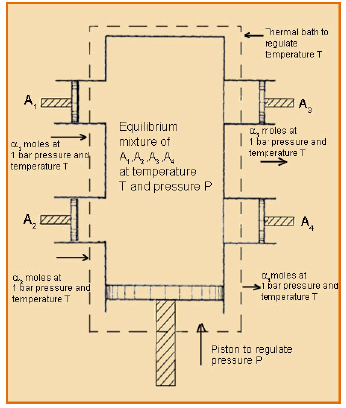Fig. 8.2 Apparatus in which a gas-phase reaction occurs at equilibrium (van't Hoff equilibrium box)

temperature of 2980  K Using the sign convention adopted above, the standard enthalpy of reaction at any temperature T may be mathematically expressed as follows: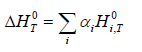...(8.2)

Where,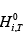is the standard state enthalpy of species ‘i’ at the temperature T, and the summation is over all the reactants and products. For example, on expansion the eqn. 8.2 takes the following form for the reaction depicted in eqn. 8.1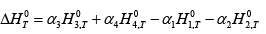...(8.2)

If we further consider that each molecular species ‘i’ is formed from j elements each, an expression for the standard enthalpy of formation results: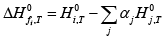...(8.3)

Where, the summation is over all j constituent elements that make up the ith molecule, ∆H 0fi ,T is standard state enthalpy of formation of the ith molecule at T, and H 0j ,T the standard state enthalpy of the jth atomic species. If all H 0j ,T are arbitrarily set to zero as the basis of calculation then eqn. 8.3 simplifies to: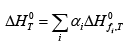...(8.4)

In such a case eqn. 1 becomes: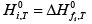...(8.5)

Values of Standard Enthalpy of formation of select substances are shown in Appendix VIII. For simplicity in the subsequent equations we drop the subscript T, but implicitly all terms correspond to temperature T. Now writing H i0 in a differential form: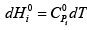...(8.6)

Where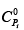is the specific heat of the ith species corresponding to its standard state. Note that since the standard state pressure for all substances is 1 bar in terms of pressure, for gases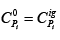, while for liquids and solids it is the actual value of the specific heat at 1 bar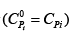Since the specific heat of liquids and solids are weakly dependent on pressure, it helps write eqn. 8.6 in the general form shown. The following summation may be applied on eqn. 8.6 to give:...(8.7)

Since each ξi is constant one may write: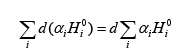...(8.8)

Or: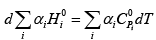...(8.9)

Thus: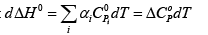...(8.10)

Where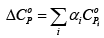Thus on integrating eqn. 8.10, between a datum Tand any T, we have: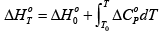...(8.11)

Note that since the standard state pressure is always at 1 bar, for all species one may write the general form of relation for specific heat capacity: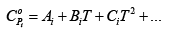...(8.12)

(The values of and CPoi thus are those shown in Appendix III).

Eqn. 8.12 may be substituted in eqn. 8.11 which leads to: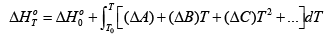...(8.13)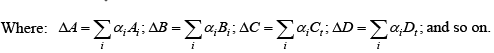The standard enthalpy of reaction is most often reported at 2980K. Using this value as the datum, the value of the standard heat of reaction at any other temperature can be evaluated using eqn. 8.13. As evident from eqn. 8.5 the enthalpy of a reaction may be recovered from the enthalpy of formation of the individual species for a reaction.

In continuance of the foregoing considerations one may also define a standard Gibbs free energy change of a reaction. As we will see in the later sections, this property is essential to computing the equilibrium constant for a reaction at any temperature. As with enthalpy of reaction (eqn. 8.2) the standard Gibbs free energy change at any temperature is given by the function: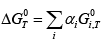...(8.14)

Thus,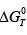is the difference between the Gibbs energies of the products and reactants when each is in its standard state as a pure substance at the system temperature and at a fixed pressure. Thus, just as the standard enthalpy of reaction is dependent only on temperature (the standard state pressure being fixed by definition), so is the Gibbs free energy change of a reaction. It follows that when the temperature is fixedis independent of the reaction pressure or composition. Indeed extending the argument, one can define any standard property change of reaction by the same expression; all being functions of temperature alone: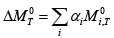...(8.15)

Where: M ≡ U , H , S, A,G.

In the context of chemical reaction equlibria the relations between the standard enthalpy of reaction and the standard Gibbs energy change of reaction is of particular significance. Using the form described by eqn. 5.31, since any standard property change of a reaction is only temperature dependent, one may write: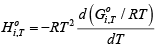...(8.16)

Multiplying of both sides of this equation byα i and summing over all species one obtains: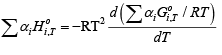This may be written as: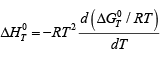...(8.17)

Or: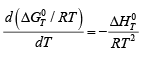...(8.18)

Now substituting eqn. 8.13 in 8.18: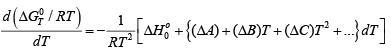If we know the standard Gibbs free energy changeat a particular temperature T0 (typically, values are reported at 2980K the above equation may be integrated as follows: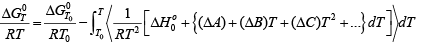...(8.19)

Or finally: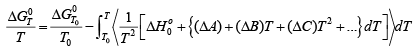...(8.20)

The document Standard Enthalpy and Gibbs Free Energy of Reaction Notes | Study Additional Documents & Tests for Civil Engineering (CE) - Civil Engineering (CE) is a part of the Civil Engineering (CE) Course Additional Documents & Tests for Civil Engineering (CE).
All you need of Civil Engineering (CE) at this link: Civil Engineering (CE)Use Code STAYHOME200 and get INR 200 additional OFF

## Additional Documents & Tests for Civil Engineering (CE)

62 docs|6 tests

Track your progress, build streaks, highlight & save important lessons and more!

,

,

,

,

,

,

,

,

,

,

,

,

,

,

,

,

,

,

,

,

,

;